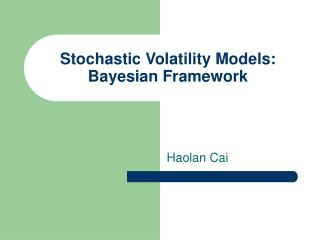Download PresentationStochastic Volatility Models: Bayesian Framework

Loading in 2 Seconds...

# Stochastic Volatility Models: Bayesian Framework - PowerPoint PPT Presentation

Stochastic Volatility Models: Bayesian Framework. Haolan Cai. Introduction. Idea: model returns using the volatility Important: must capture the persistence of the volatilities (i.e. volatility clusters) along with other characteristicsI am the owner, or an agent authorized to act on behalf of the owner, of the copyrighted work described.
Download Presentation## Stochastic Volatility Models: Bayesian Framework

An Image/Link below is provided (as is) to download presentation

Download Policy: Content on the Website is provided to you AS IS for your information and personal use and may not be sold / licensed / shared on other websites without getting consent from its author.While downloading, if for some reason you are not able to download a presentation, the publisher may have deleted the file from their server.

- - - - - - - - - - - - - - - - - - - - - - - - - - E N D - - - - - - - - - - - - - - - - - - - - - - - - - -
Presentation Transcript
1. Introduction Idea: model returns using the volatility Important: must capture the persistence of the volatilities (i.e. volatility clusters) along with other characteristics Use: a class of Hidden Markov Models (HMM) known as Stochastic Volatility Models (SV models)

2. Basic Model Where θ = (φ, v) is the parameter space for the AutoRegressive process of order 1 (i.e. linear). φ is the persistence of the model.

3. Transformation of Model Previous model is non-linear which creates complications. When we apply the following transformation: We get nice linear form: Where is the error term with the following form:

4. The Problem Child does not have a close form from which it is easy to sample. However it can be accurately approximated with a discrete mixture of normals. In this case the optimal J is equal to 7. Kim, Shephard and Chib (1998)

5. Bayesian Framework Now all the parameters have nice distributions from which they can be sampled using a Gibbs sampling algorithm. Use semi-informative priors (above) with parameters loosely developed from data. Imposes some but little structure to the sampling. The algorithm was ran for 500 iterations with a burn in period of 50.

6. The Problem Child (again) In order to sample we sample from the mixture of normals. This is done by a Forward Filtering, Backwards Sampling (FFBS) algorithm. A Kalman filter is applied from t = 0 to t = n. Then the states (xn, xn-1 … x0) are simulated in the backwards order. The reasoning for this more complicated sampling measure is the high AR dependence of this type of data. φ is close to 1.

7. Initial Conditions For the mixture of normals, 7 normals are chosen to fix the log chi-squared distribution. For the other parameters, initial values were chosen to sufficiently cover the parameter space as to be semi-informative but not restrictive. For example, parameters for μ are g and G; where g is the mean and G the standard deviation. Here there are chosen to be 0 and 9 respectively.

8. Data 1-minute prices from General Electric and Intel Corporation • GE: April 9, 2007 9:35 am to Jan 24, 2008 3:59 pm • Used Daily Returns for SV model

9. Checking Autocorrelation Structure

10. Results φ is steady around .956

11. Results: μ = .0037

12. Results: ν = .4150

13. Results:

14. Further Analysis Try to build in autoregressive of high order. Allow J, the number of normals used to fit the error term, to vary. What kind of predictive value does this model produce for stock returns? Does using higher frequency data improve predictive and/or fit value?